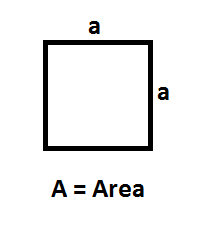# Area Formulas

Area is the size of a two-dimensional surface. It is defined as the amount of two-dimensional space occupied by an object. Area formulas have many practical applications in building, farming, architecture, science. The area of a shape can be determined by placing the shape over a grid and counting the number of squares that covers the entire space. For example, the area of a square can be calculated using
$$\begin{array}{l}a^{2}\end{array}$$
where a is the length of its side.List of Formulas

 Figures Formula Variables Rectangle Area = l $$\begin{array}{l}\times\end{array}$$ w l =  length w  = width Square Area  = $$\begin{array}{l}a^{2}\end{array}$$ a = sides of square Triangle Area = $$\begin{array}{l}\frac{1}{2}\end{array}$$bh b = base h = height Circle Area = $$\begin{array}{l}\pi\end{array}$$$$\begin{array}{l}r^{2}\end{array}$$ r = radius of circle Trapezoid Area =$$\begin{array}{l}\frac{1}{2}\end{array}$$(a + b)h a = base 1 b = base 2 h = vertical height Ellipse Area = $$\begin{array}{l}\pi\end{array}$$ab a = radius of major axis b = radius of minor axis